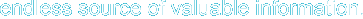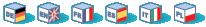Next Previous Contents

## 6. Conditionals

Conditionals let you decide whether to perform an action or not, this decision is taken by evaluating an expression.

## 6.1 Dry Theory

Conditionals have many forms. The most basic form is: if expression then statement where 'statement' is only executed if 'expression' evaluates to true. '2<1' is an expresion that evaluates to false, while '2>1' evaluates to true.xs

Conditionals have other forms such as: if expression then statement1 else statement2. Here 'statement1' is executed if 'expression' is true,otherwise 'statement2' is executed.

Yet another form of conditionals is: if expression1 then statement1 else if expression2 then statement2 else statement3. In this form there's added only the "ELSE IF 'expression2' THEN 'statement2'" which makes statement2 being executed if expression2 evaluates to true. The rest is as you may imagine (see previous forms).

The base for the 'if' constructions in bash is this:

if [expression];

then

code if 'expression' is true.

fi

## 6.2 Sample: Basic conditional example if .. then

``` #!/bin/bash if [ "foo" = "foo" ]; then echo expression evaluated as true fi ```

The code to be executed if the expression within braces is true can be found after the 'then' word and before 'fi' which indicates the end of the conditionally executed code.

## 6.3 Sample: Basic conditional example if .. then ... else

``` #!/bin/bash if [ "foo" = "foo" ]; then echo expression evaluated as true else echo expression evaluated as false fi ```

## 6.4 Sample: Conditionals with variables

``` #!/bin/bash T1="foo" T2="bar" if [ "\$T1" = "\$T2" ]; then echo expression evaluated as true else echo expression evaluated as false fi ```

Next Previous Contents

Hosting by: Hurra Communications Ltd.
Generated: 2007-01-26 17:57:44